# Class 12 Maths NCERT Solutions for Chapter 12 Linear Programming Exercise 12.1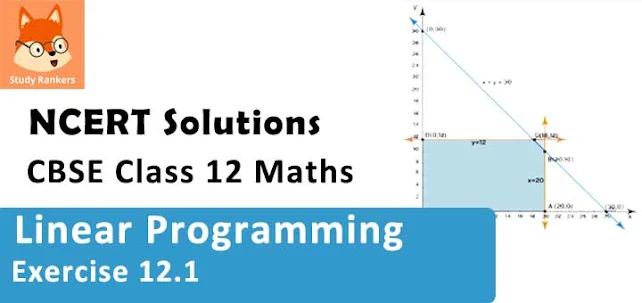### Linear Programming Exercise 12.1 Solutions

1. Solve the following Linear Programming Problems graphically:
Maximise Z = 3x + 4y
subject to the constraints : x + y ≤ 4, x ≥ 0, y ≥ 0.

Solution

The feasible region determined by the constraints, x y ≤ 4, x ≥ 0, y ≥ 0, is as follows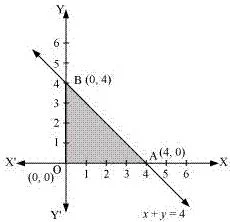The corner points of the feasible region are O (0, 0), A (4, 0), and B (0, 4). The values of Z at these points are as follows.

 Corner point Z = 3x + 4y O(0, 0) 0 A(4, 0) 12 B(0, 4) 16 → Maximum

Therefore, the maximum value of Z is 16 at the point B (0, 4).

2. Solve the following Linear Programming Problems graphically:
Minimise  Z = – 3x + 4 y subject to x + 2y ≤ 8, 3x + 2y ≤ 12, x ≥ 0, y ≥ 0.

Solution

The feasible region determined by the system of constraints, x + 2y ≤ 8, 3x + 2y ≤ 12. x ≥ 0, and y ≥ 0, is as follows.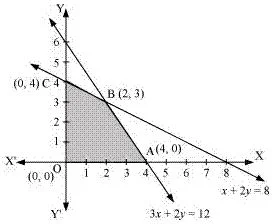The corner points of the feasible region are O (0, 0), A (4, 0), B (2, 3), and C (0, 4).
The values of Z at these corner points are as follows.

 Corner point Z = −3x + 4y 0(0, 0) 0 A(4, 0) −12 → Minimum B(2, 3) 6 C(0, 4) 16

Therefore, the minimum value of Z is −12 at the point (4, 0).

3. Solve the following Linear Programming Problems graphically:
Maximise Z = 5x + 3y subject to 3x + 5y ≤ 15, 5x + 2y ≤ 10, x ≥ 0, y ≥ 0

Solution

The feasible region determined by the system of constraints, 3x + 5y ≤ 15,
5x + 2y ≤ 10, x ≥ 0, and y ≥ 0, are as follows.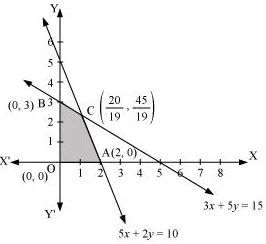The corner points of the feasible region are O(0, 0), A(2, 0), B(0, 3) and C(20/19 , 45/19).
The values of Z at these corner points are as follows.

 Corner point Z = 5x + 3y 0(0, 0) 0 A(2, 0) 10 B(0, 3) 9 C(20/19,45/19) 235/19 → Maximum

Therefore, the maximum value of Z is 235/19 at the point (20/19, 45/19).

4. Solve the following Linear Programming Problems graphically:
Minimise Z = 3x + 5y such that x + 3y ≥ 3, x + y ≥ 2, x, y ≥ 0.

Solution

The feasible region determined by the system of constraints, x + 3y ≥ 3, x + y ≥ 2, and x, y ≥ 0, is as follows.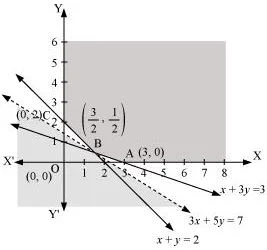It can be seen that the feasible region is unbounded.
The corner points of the feasible region are A (3, 0), B (3/2, 1/2), and C (0, 2).
The values of Z at these corner points are as follows.

 Corner point Z = 3x + 5y A(3, 0) 9 B (3/2, 1/2) 7 → Smallest C(0, 2) 10

As the feasible region is unbounded, therefore, 7 may or may not be the minimum value of Z.
For this, we draw the graph of the inequality, 3x + 5y < 7, and check whether the resulting half plane has points in common with the feasible region or not.
It can be seen that the feasible region has no common point with 3x + 5y < 7 Therefore, the minimum value of Z is 7 at (3/2, 1/2) .

5. Solve the following Linear Programming Problems graphically:
Maximise Z = 3x + 2y subject to x + 2y ≤ 10, 3x + y ≤ 15, x, y ≥ 0.

Solution

The feasible region determined by the constraints, x + 2y ≤ 10, 3x + ≤ 15, x ≥ 0, and y ≥ 0, is as follows.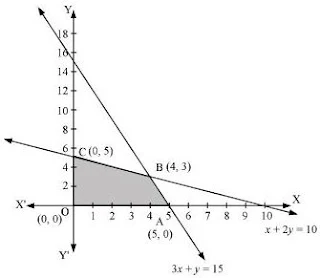The corner points of the feasible region are A (5, 0), B (4, 3), and C (0, 5).
The values of Z at these corner points are as follows.

 Corner point Z = 3x + 2y A(5, 0) 15 B(4, 3) 18 → Maximum C(0, 5) 10

Therefore, the maximum value of Z is 18 at the point (4, 3).

6. Solve the following Linear Programming Problems graphically:
Minimise  Z = x + 2y subject to 2x + y ≥ 3, x + 2y ≥ 6, x, y ≥ 0.

Solution

The feasible region determined by the constraints, 2x + y ≥ 3, x + 2y ≥ 6, x ≥ 0, and y ≥ 0, is as follows.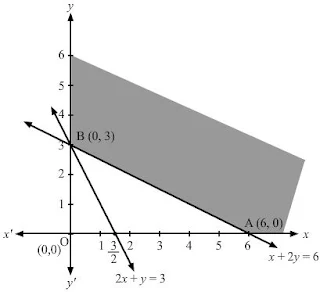The corner points of the feasible region are A (6, 0) and B (0, 3).
The values of Z at these corner points are as follows.

 Corner point Z = x + 2y A(6, 0) 6 B(0, 3) 6

It can be seen that the value of Z at points A and B is same. If we take any other point such as (2, 2) on line x + 2y = 6, then Z = 6
Thus, the minimum value of Z occurs for more than 2 points.
Therefore, the value of Z is minimum at every point on the line, x + 2y = 6

7. Show that the minimum of Z occurs at more than two points.
Minimise and Maximise Z = 5x + 10 y subject to x + 2y ≤ 120, x + y ≥ 60, x – 2y ≥ 0, x, y ≥ 0.

Solution

The feasible region determined by the constraints, x + 2y ≤ 120, x + y ≥ 60, x − 2y ≥ 0, x ≥ 0, and y ≥ 0, is as follows.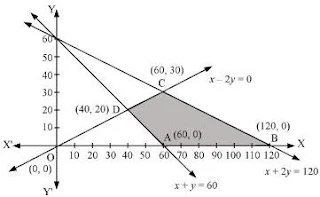The corner points of the feasible region are A (60, 0), B (120, 0), C (60, 30), and D (40, 20).
The values of Z at these corner points are as follows.

 Corner point Z = 5x + 10y A(60, 0) 300 → Minimum B(120, 0) 600 → Maximum C(60, 30) 600 → Maximum D(40, 20) 400

The minimum value of Z is 300 at (60, 0) and the maximum value of Z is 600 at all the points on the line segment joining (120, 0) and (60, 30).

8. Show that the minimum of Z occurs at more than two points
Minimise and Maximise Z = x + 2y subject to x + 2y ≥ 100, 2x – y ≤ 0, 2x + y ≤ 200; x, y ≥ 0.

Solution

The feasible region determined by the constraints, x + 2y ≥ 100, 2x − y ≤ 0, 2x + ≤ 200, x ≥ 0, and y ≥ 0, is as follows.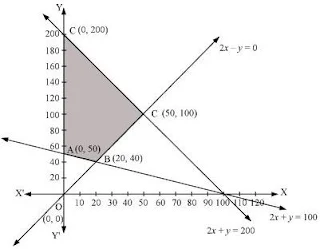The corner points of the feasible region are A(0, 50), B(20, 40), C(50, 100), and D(0, 200).
The values of Z at these corner points are as follows.

 Corner point Z = x + 2y A(0, 50) 100 → Minimum B(20, 40) 100 → Minimum C(50, 100) 250 D(0, 200) 400 → Maximum

The maximum value of Z is 400 at (0, 200) and the minimum value of Z is 100 at all the points on the line segment joining the points (0, 50) and (20, 40).

9. Show that the minimum of Z occurs at more than two points
Maximise Z = – x + 2y, subject to the constraints x ≥ 3, x + y ≥ 5, x + 2y ≥ 6, y ≥ 0.

Solution

The feasible region determined by the constraints, x ≥ 3, x + y ≥ 5, x + 2y ≥ 6, and y ≥ 0, is as follows.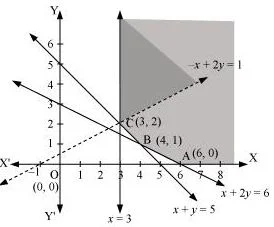It can be seen that the feasible region is unbounded.
The values of Z at corner points A (6, 0), B (4, 1), and C (3, 2) are as follows.

 Corner point Z = −x + 2y A(6, 0) Z = − 6 B(4, 1) Z = − 2 C(3, 2) Z = 1

As the feasible region is unbounded, therefore, Z = 1 may or may not be the maximum value.
For this, we graph the inequality, −x + 2y > 1, and check whether the resulting half plane has points in common with the feasible region or not.
The resulting feasible region has points in common with the feasible region.
Therefore, Z = 1 is not the maximum value. Z has no maximum value.

10. Show that the minimum of Z occurs at more than two points.
Maximise Z = x + y, subject to x – y ≤ –1, –x + y ≤ 0, x, y ≥ 0.

Solution

The region determined by the constraints, x - y ≤ -1, -x + y ≤0, x, y ≥ 0, is as follows.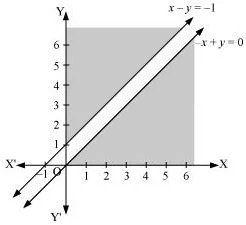There is no feasible region and thus, Z has no maximum value.